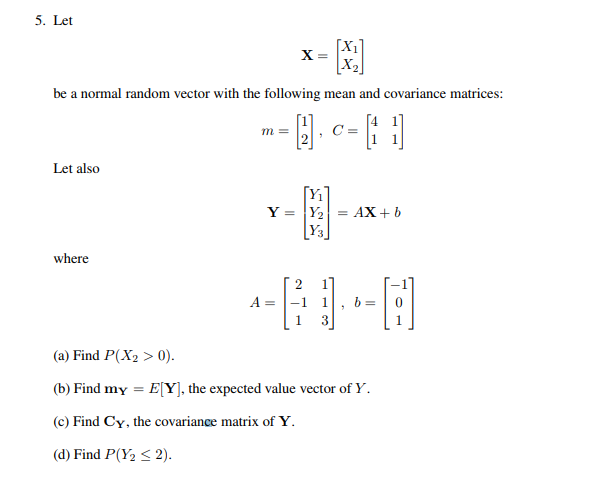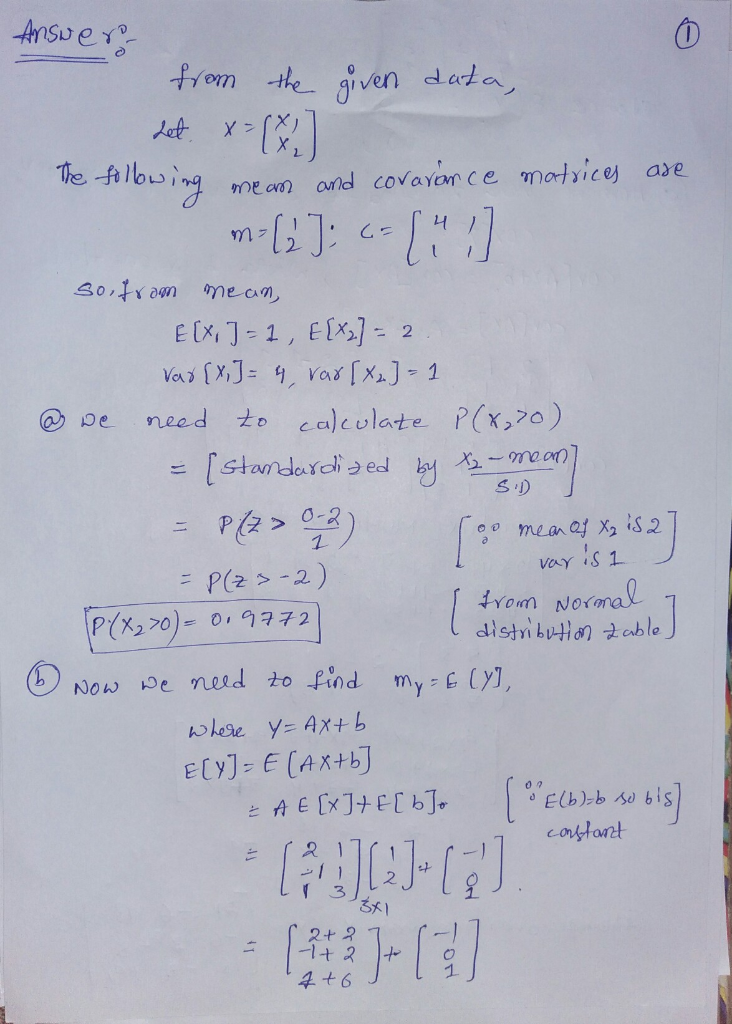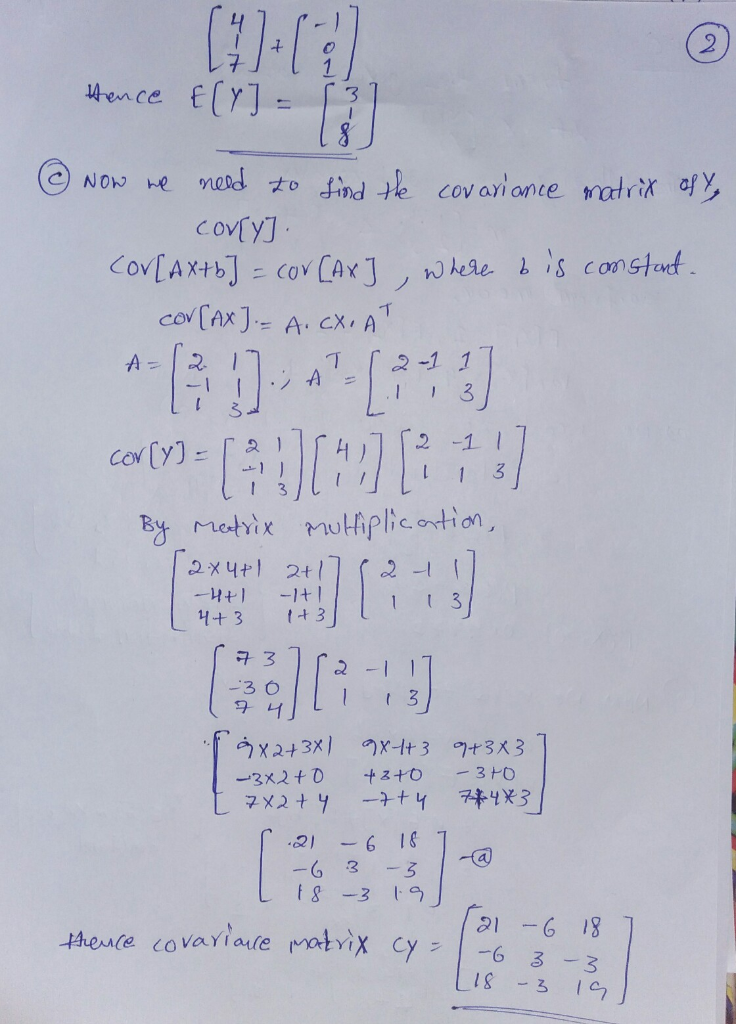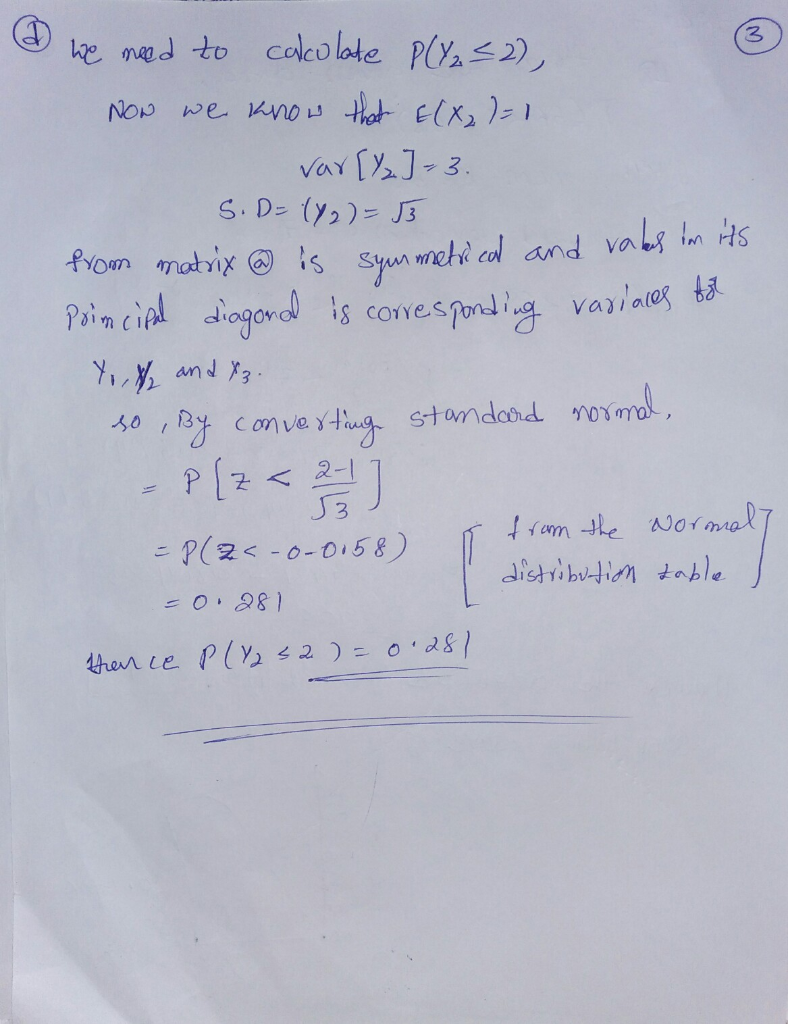Homework Help Question & Answers

# 5. Let be a normal random vector with the following mean and covariance matrices: 2 Let also Y; Y...5. Let be a normal random vector with the following mean and covariance matrices: 2 Let also Y; Y3 where (a) Find P(X2 >0). b Find my EY]. the expected value vector of Y. (c) Find CY, the covariance matrix of Y d) Find P(Y 2).##### Add Answer of: 5. Let be a normal random vector with the following mean and covariance matrices: 2 Let also Y; Y...
More Homework Help Questions Additional questions in this topic.

• #### Let S be the sphere r2 + y2 + z2-k2 oriented outward and let F be the vector field (r, y, 2)/(a2 +y2 +2/2. Find (i) the normal vector field n on S (ii) the normal component of F on S and (ii) the flu...

Need Online Homework Help?

Get FREE EXPERT Answers
WITHIN MINUTES
Related Questions